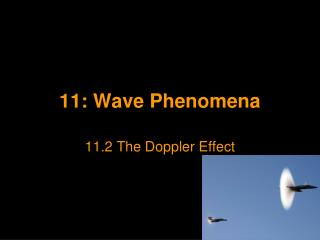DownloadDownload Presentation11: Wave Phenomena

# 11: Wave Phenomena

Download Presentation## 11: Wave Phenomena

- - - - - - - - - - - - - - - - - - - - - - - - - - - E N D - - - - - - - - - - - - - - - - - - - - - - - - - - -
##### Presentation Transcript

1. 11: Wave Phenomena 11.2 The Doppler Effect

2. The Doppler Effect When a car passes you on the street, the frequency of its engine note appears to change. This is due to the ‘Doppler effect’: It can occur due to any relative motion; i.e. Either due to the source or the observer moving. The Doppler effect is the change in observed frequency of a wave due to relative motion between the wave source and an observer.

3. Source MovingLink 1 - Link 2 - Doppler / Sound A source of sound S, moving to the right at speed u, emitting waves of speed v and frequency f0. An observer at X will detect waves of different frequency f1: S S X X

4. It takes time t for the waves to reach X, the distance between the source and observer after this time (SX) must be: distance = distance travelled by waves – distance travelled by car so... distance = vt - ut = (v – u) t In this time the number of waves produced by the source = f0 t So wavelength of these detected waves appears to be... λ1 = distance / number of waves λ1 = (v - u) t / f0 t λ1 = v - u / f0 If v = fλ then observed frequency f1 = v / λ1 so... f1 = v f0 v - u

5. We can similarly prove that for an observer behind the source of sound waves the total distance travelled by the waves appears to have increased to (v + u)t . Hence... f2 = v f0 v + u In both these cases the change in frequency is as a result of the wavelength appearing to change as the source moves.

6. E.g. A Ferrari engine emits sound waves of frequency 80.00Hz. How fast must the Ferrari go for the observed frequency to increase beyond the human range of hearing (speed of sound = 330.0 ms-1)? A u = 328.7 ms-1

7. Observer Moving (The wavelength does not change.) If the observer moves, this results in the velocity of the sound appearing to change. Y S Z

8. For an observer at Y moving at speed u towards the source of sound S, the observed frequency f1 is given by... f1 = (v + u) / λ (velocity increases) But the wavelength of the emitted sound is given by...λ = v / f0 So... And for an observer at Z moving away from the source of sound... f1 = (v + u) f0 v f2 = (v - u) f0 v

9. E.g. Calculate the frequency heard by the driver of a (silent!) car moving at 30.0ms-1away from a sound of frequency 750Hz. A f2 = 682 Hz

10. EM Radiation and the Doppler Effect As the speed of light cannot change, it can be shown that for EM radiation such as light, the change in frequency (or ‘Doppler shift’) is given by... Where... Δf = change in frequency (Hz) v = relative speed of the source and observer (ms-1) c = speed of light f0 = emitted frequency (Hz) Δf = v f0 c Note: This formula is an approximation and can only be used if v << c

11. E.g.(You need to be able to remember this example) A ‘Gatso’ speed camera emits microwaves of wavelength 2.6cm. These are reflected off a car moving at 160kmh-1. Calculate the change in the frequency (Doppler shift) that would be detected in the car. If the wave is then reflected, calculate the total Doppler shift in the frequency detected back at the Gatso camera. a. Δf= 1700Hz b. Δf= 3400Hz

12. Subtitle Text

13. Subtitle Text

14. A. B. C. D. Subtitle Text

15. Subtitle Text S

16. Subtitle Text

17. Subtitle Text

18. Subtitle Text

19. Subtitle Text

20. Subtitle Text

21. Subtitle Text

22. Subtitle Text